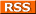Comments Rss
PostGIS MakePoint

# Creating Point Geometries with ST_MakePoint

There are numerous ways of creating point geometries in PostGIS. We have covered these ways in other snippets. Check out the fulling links for other examples.

ST_MakePoint is perhaps in terms of speed the fastest way of creating a PostGIS geometry and on tests I have done, can be as much as 5 to 10 times faster. The downside of MakePoint is that it is not defined in the OGC spec so its not quite as portable across other spatial databases as is ST_GeomFromText and PointFromText.

ST_MakePoint is used in the form MakePoint(x, y) which for pretty much all spatial reference systems means ST_MakePoint(longitude, latitude)

## Transformable (Reprojectable) and Non-Transformable Geometries

MakePoint used alone creates a nontransformable geometry (one that has an SRID = -1, so because of that, MakePoint is usually used in conjunction with SetSRID to create a point geometry with spatial reference information. Examples below and the EWKT output to demonstrate the differences.

### Example below can not be used in a transformation to transform to another Spatial Reference System

```SELECT ST_MakePoint(-71.09405383923, 42.3151215523721) as the_point, AsEWKT(ST_MakePoint(-71.09405383923, 42.3151215523721)) as ewkt_rep ``` Note - the ewkt_rep output looks exactly like the WKT output - e.g. no spatial reference information so can not be passed into a transformation call - POINT(-71.09405383923 42.3151215523721)

### Emparting Spatial Information to MakePoint Geometry

```SELECT SETSRID(ST_MakePoint(-71.09405383923, 42.3151215523721),4326) as the_point, ST_AsEWKT(ST_SetSRID(ST_MakePoint(-71.09405383923, 42.3151215523721),4326)) as ewkt_rep ```
Note - the ewkt_rep output looks like the regular WKT output but has extra information about the spatial refence system the point is defined in SRID=4326;POINT(-71.09405383923 42.3151215523721)